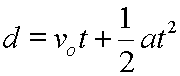## StatsMiniBlog: Significance tests. Step three.So, many of you will know that the first rule is that the Doctor lies. The last post might have given you the impression that that was the whole of statistics … but there is a bit more. The first idea that goes beyond the simple question is ‘how are these two continuous variables related to each other’?

This isn’t too tricky to grasp. It’s correlation. How does shoe size relate to height? Are the two things related?

The values that the ‘statistical machinery’ produces are generally two fold: a p-value and a correlation coefficient.

Now the p-value still gives us an answer to the question ‘what’s the likelihood that the results I’ve got from these two groups are different only because of the play of chance?’. So if this is ‘significant’ it does not mean that one variable describes the other really well, it just means that the two things are highly unlikely to be just a chance finding.

The correlation coefficient (r) gives us an idea about how closely the two things are related. A value of +1 means perfectly related, as one variable increases, the other variable does too. A value of  -1 means a perfect inverse relationship – as one increases, the other decreases. In many ways, it’s this value, not the p-value, that is the one we’re usually looking for to explain what’s going on.(In fact, we  might want to know even more than just the correlation coefficient… keep reading for future blog posts …)

One quick final bit; which test machinery to use. For two sets of Normal variables, it’s the Pearson correlation coefficient. For non-Normal data, it’s generally Spearman’s rank correlation coefficient.

– Archi

(Visited 147 times, 1 visits today)